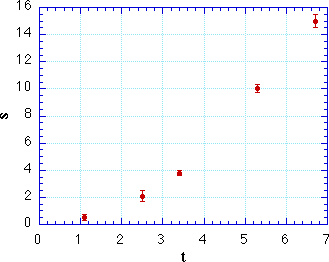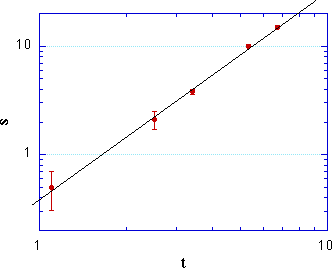Understanding Basic StatisticsFittingExerciseExcelIgorKaleidagraphOriginPower LawsDimensional Analysis

# Power Law

When one quantity (say y) depends on another (say x) raised to some power, we say that y is described by a power law. For example, the distance traveled by an object in free fall (in the absence of air resistance) is given by

s(t) = 1/2 gt2        (1)Ignoring the dependence on g, this equation says that s is proportional to the square of t. Plotting on linear (Cartesian) axes produces a parabola. Now, a parabola is a very beautiful curve, but it is difficult to eyeball a curve and say with confidence that it is a parabola. It is even harder to eyeball some data points, with their uncertainties, and tell whether they follow a parabolic curve. Eyeballs are much happier with straight lines.

There is a handy way to convert this power-law relationship into a straight line; just take the logarithm of both sides of the equation:

log(s) = 2 log(t) + log(1/2 g)         (2)If we plot log(s) on the y axis and log(t) on the x axis, we get a straight line with slope 2 (which is the exponent of t). Using Kaleidagraph or Origin you can just double-click the axes to convert the display from linear to logarithmic. You can also perform a proper c2 fit, as described on the Fitting page.
Understanding Basic StatisticsFittingExerciseExcelIgorKaleidagraphOriginPower LawsDimensional AnalysisCopyright © Harvey Mudd College Physics Department 241 Platt Blvd., Claremont, CA 91711 909-621-8024 http://www.physics.hmc.edu/ WebMaster (at) physics.hmc.edu Last modified: 26 August 2012